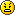Page 1 of 1

### Converting Excel formula into LC

Posted: Fri Apr 19, 2013 4:11 am
Hello,
I'm trying to change this code:

Code: Select all

``MOD(DEGREES(ACOS(((SIN(RADIANS(B3))*COS(RADIANS(AD2)))-SIN(RADIANS(T2)))/(COS(RADIANS(B3))*SIN(RADIANS(AD2)))))+180,360)``
Into an LC function.

When I thought I had it right the results wouldn't match up to Excels results.Any help welcome.
Thank you,
Simon

### Re: Converting Excel formula into LC

Posted: Fri Apr 19, 2013 9:12 pm
Just thought I'd bump this message.

Thanks,
Simon

### Re: Converting Excel formula into LC

Posted: Sat Apr 20, 2013 5:29 am
Got It!Code: Select all

``````Excel
Simon